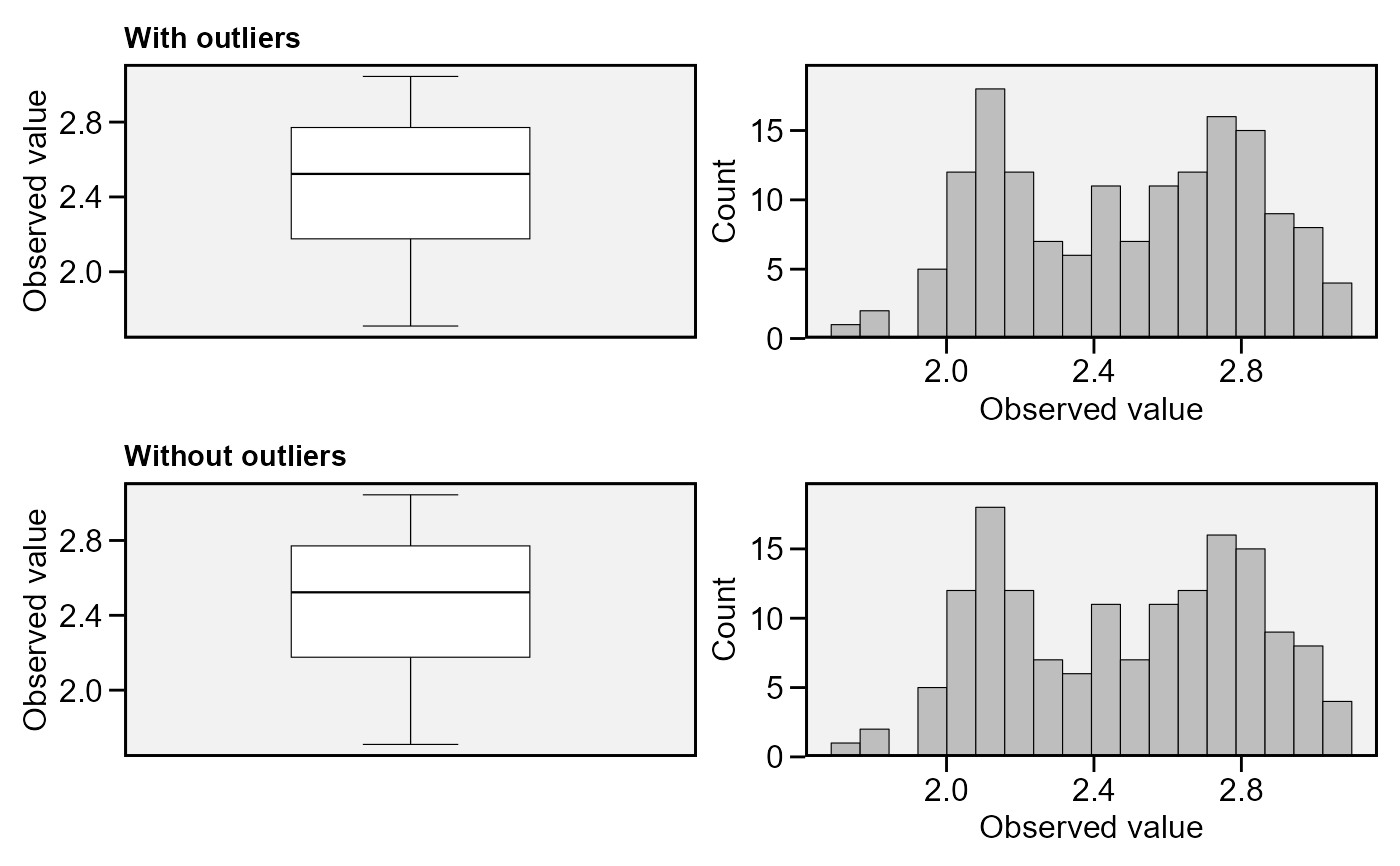## Usage

find_outliers(
.data = NULL,
var = NULL,
by = NULL,
plots = FALSE,
coef = 1.5,
verbose = TRUE,
plot_theme = theme_metan()
)

## Arguments

.data

The data to be analyzed. Must be a dataframe or an object of class split_factors.

var

The variable to be analyzed.

by

One variable (factor) to compute the function by. It is a shortcut to dplyr::group_by(). To compute the statistics by more than one grouping variable use that function.

plots

If TRUE, then histograms and boxplots are shown.

coef

The multiplication coefficient, defaults to 1.5. For more details see ?boxplot.stat.

verbose

If verbose = TRUE then some results are shown in the console.

plot_theme

The graphical theme of the plot. Default is plot_theme = theme_metan(). For more details, see ggplot2::theme().

## Author

Tiago Olivoto tiagoolivoto@gmail.com

## Examples

# \donttest{
library(metan)

find_outliers(data_ge2, var = PH, plots = TRUE)#> No possible outlier identified.
#>

# Find outliers within each environment
find_outliers(data_ge2, var = PH, by = ENV)
#> No possible outlier identified.
#>
#> No possible outlier identified.
#>
#> Trait: PH
#> Number of possible outliers: 4
#> Line(s): 7 11 14 15
#> Proportion: 11.4%
#> Mean of the outliers: 2.438
#> Maximum of the outliers: 2.766  | Line 11
#> Minimum of the outliers: 1.71  | Line 7
#> With outliers:    mean = 2.167 | CV = 10.309%
#> Without outliers: mean = 2.136 | CV = 7.377%
#>
#> No possible outlier identified.
#>
#>   ENV outliers
#> 1  A1        0
#> 2  A2        0
#> 3  A3        4
#> 4  A4        0
# }